# RD Sharma Solutions for Class 11 Chapter 2 - Relations Exercise 2.3

Exercise 2.3 of Chapter 2, discusses relations, representation of a relation (which include roster form, set-builder form, by arrow diagram, by lattice), finding the domain and range of a relation, inverse of a relation. Students who aim to top their exams are advised to practice the solutions on a daily basis by referring to RD Sharma Class 11 Solutions. Practising the textbook questions will help you in analyzing your level of preparation and knowledge of the concept. The pdf of RD Sharma Class 11 Maths can be easily downloaded from the links provided below.

## Download the Pdf of RD Sharma Solutions for Class 11 Maths Exercise 2.3 Chapter 2 – Relations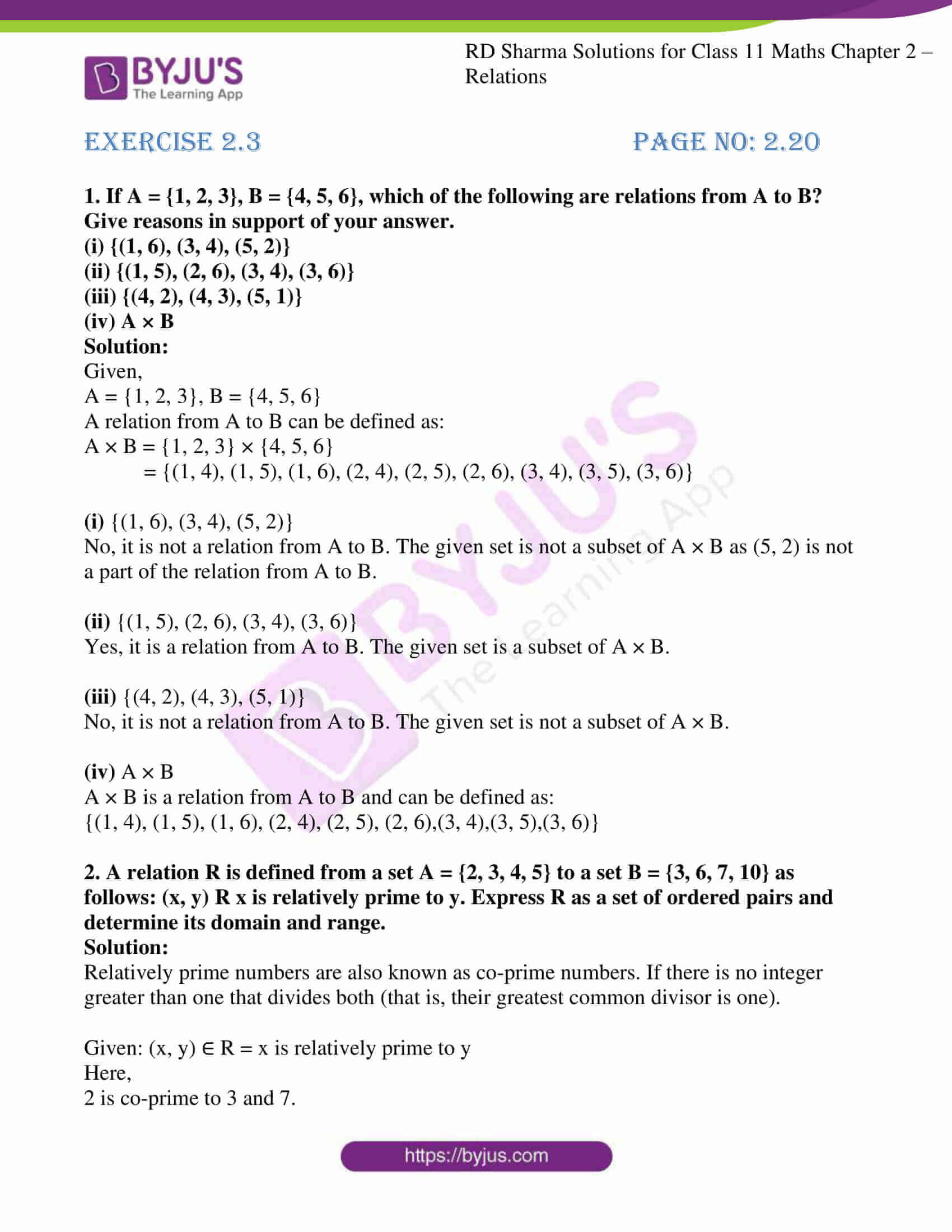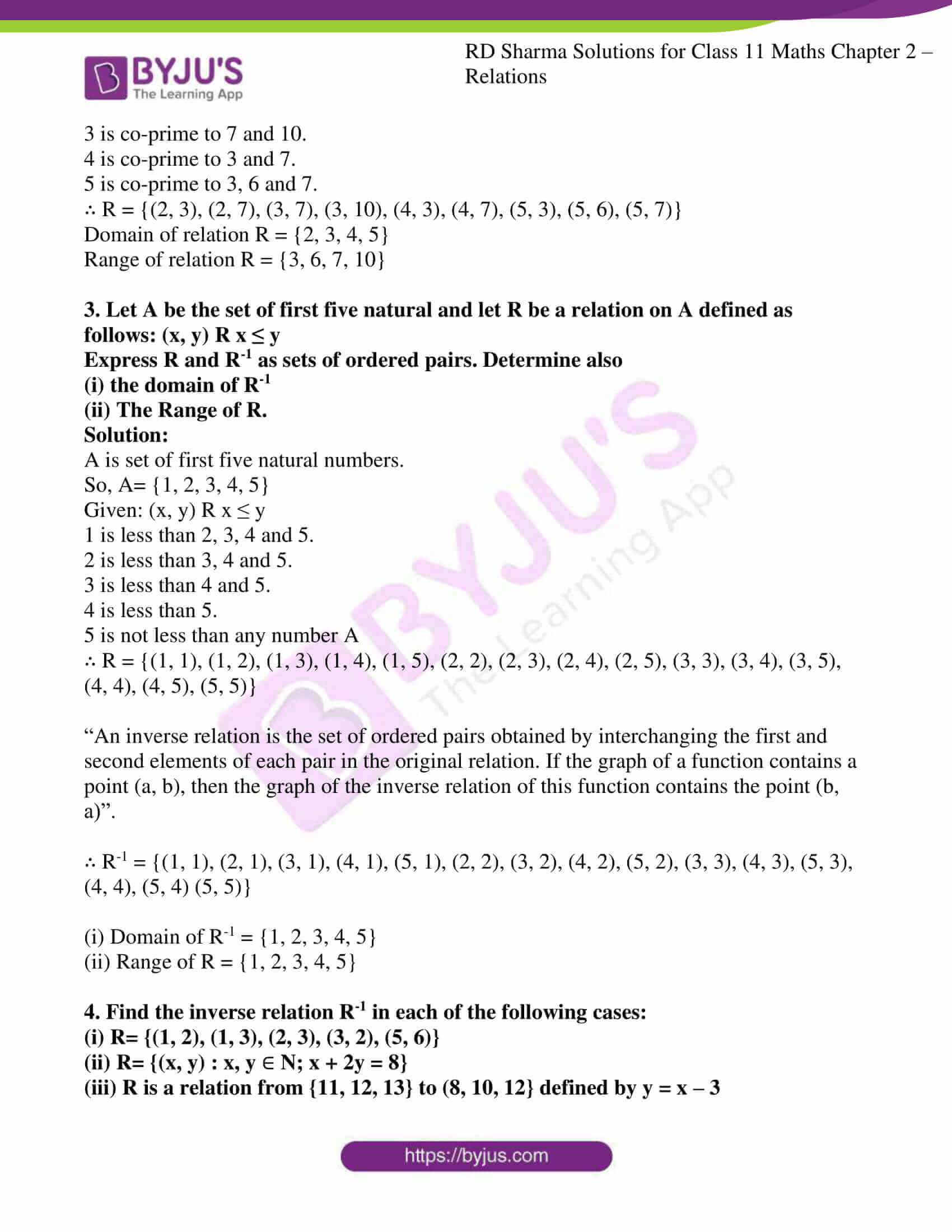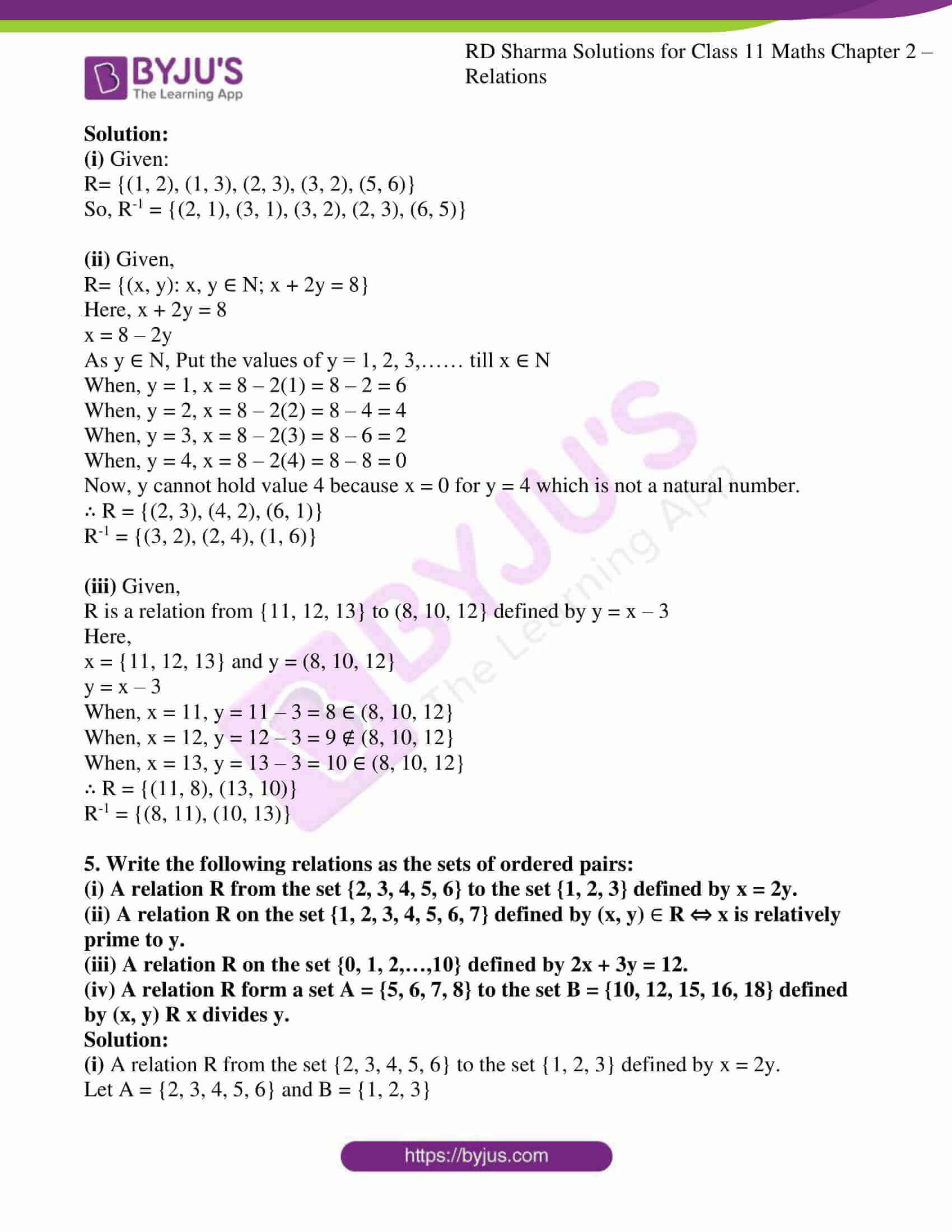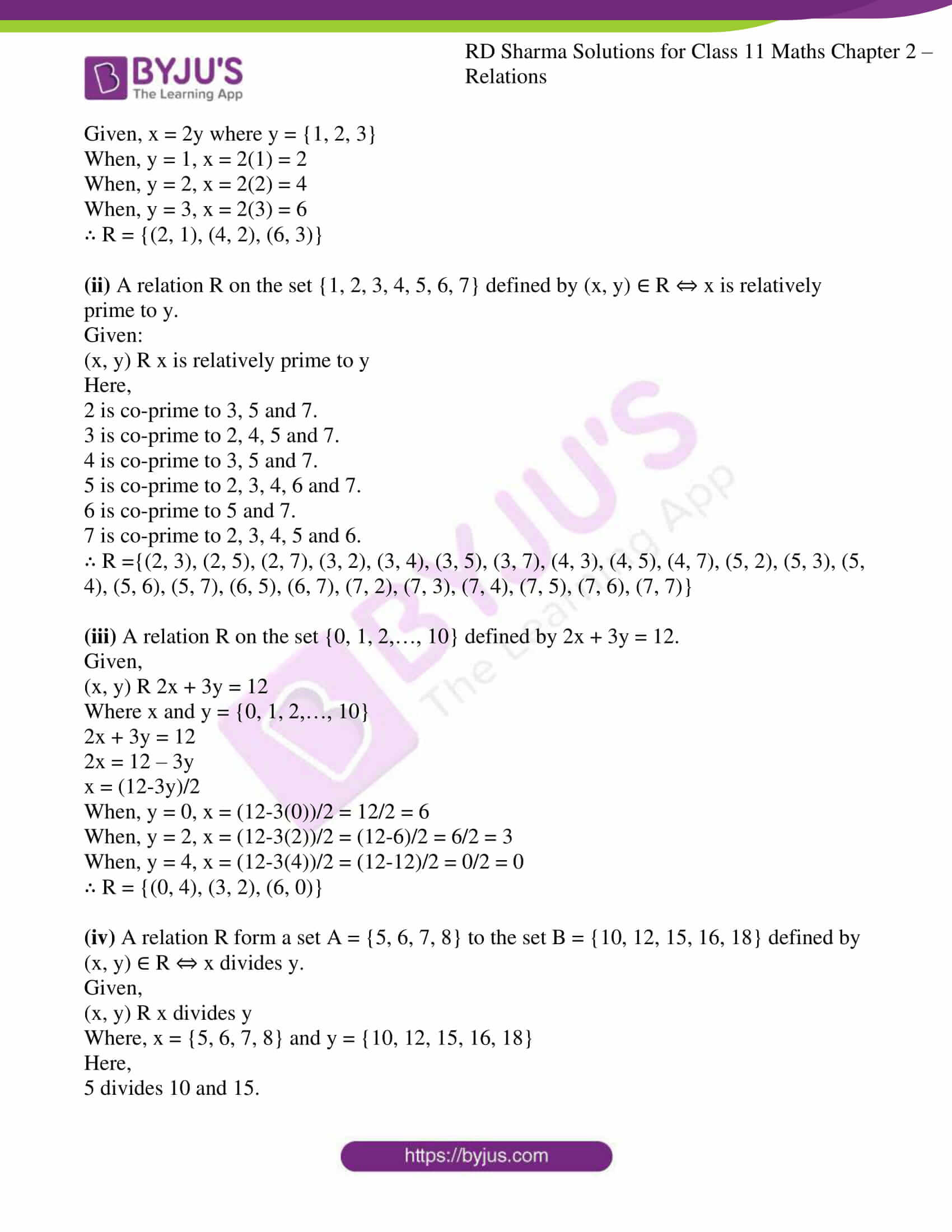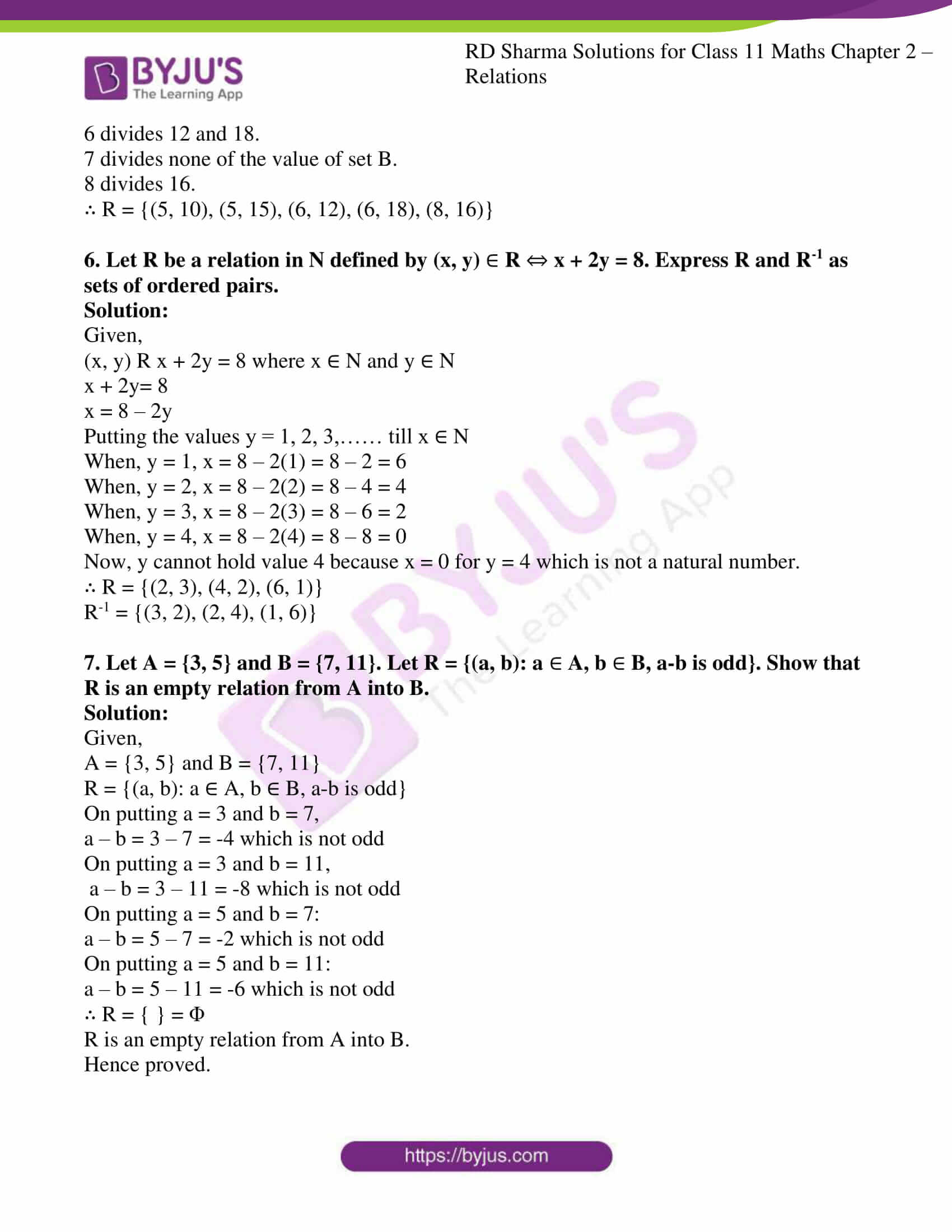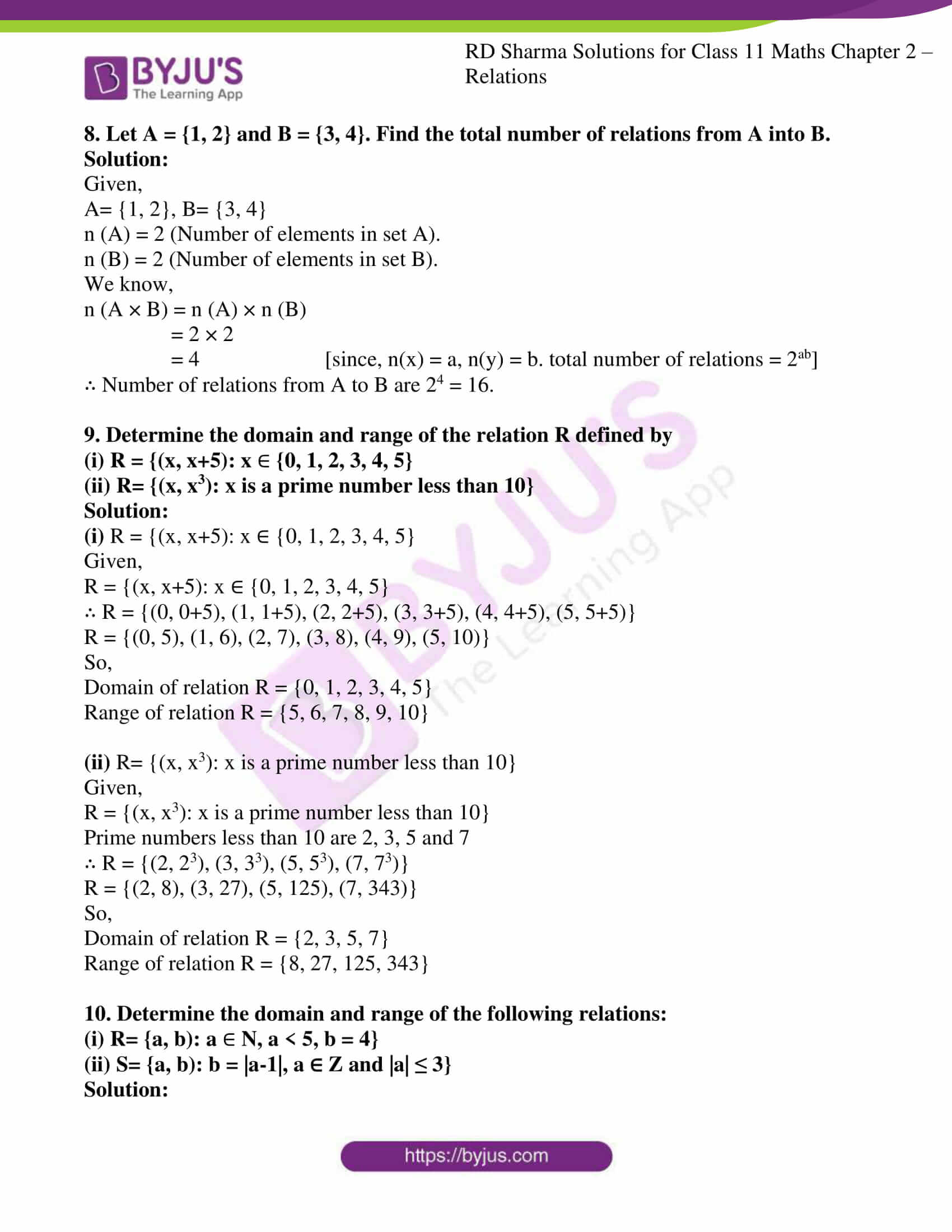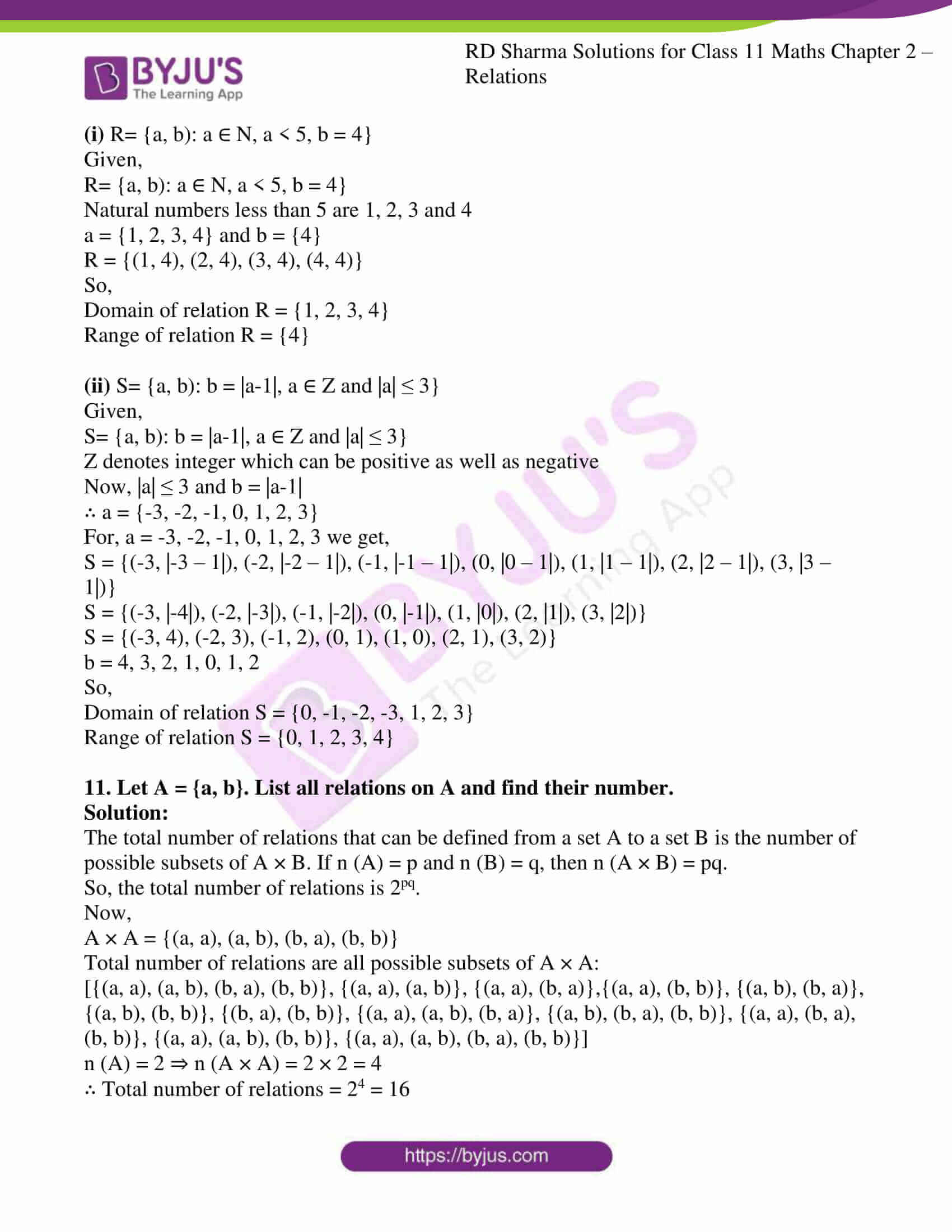### Access answers to RD Sharma Solutions for Class 11 Maths Exercise 2.3 Chapter 2 – Relations

1. If A = {1, 2, 3}, B = {4, 5, 6}, which of the following are relations from A to B?

(i) {(1, 6), (3, 4), (5, 2)}

(ii) {(1, 5), (2, 6), (3, 4), (3, 6)}

(iii) {(4, 2), (4, 3), (5, 1)}

(iv) A × B

Solution:

Given,

A = {1, 2, 3}, B = {4, 5, 6}

A relation from A to B can be defined as:

A × B = {1, 2, 3} × {4, 5, 6}

= {(1, 4), (1, 5), (1, 6), (2, 4), (2, 5), (2, 6), (3, 4), (3, 5), (3, 6)}

(i) {(1, 6), (3, 4), (5, 2)}

No, it is not a relation from A to B. The given set is not a subset of A × B as (5, 2) is not a part of the relation from A to B.

(ii) {(1, 5), (2, 6), (3, 4), (3, 6)}

Yes, it is a relation from A to B. The given set is a subset of A × B.

(iii) {(4, 2), (4, 3), (5, 1)}

No, it is not a relation from A to B. The given set is not a subset of A × B.

(iv) A × B

A × B is a relation from A to B and can be defined as:

{(1, 4), (1, 5), (1, 6), (2, 4), (2, 5), (2, 6),(3, 4),(3, 5),(3, 6)}

2. A relation R is defined from a set A = {2, 3, 4, 5} to a set B = {3, 6, 7, 10} as follows: (x, y) R x is relatively prime to y. Express R as a set of ordered pairs and determine its domain and range.

Solution:

Relatively prime numbers are also known as co-prime numbers. If there is no integer greater than one that divides both (that is, their greatest common divisor is one).

Given: (x, y) ∈ R = x is relatively prime to y

Here,

2 is co-prime to 3 and 7.

3 is co-prime to 7 and 10.

4 is co-prime to 3 and 7.

5 is co-prime to 3, 6 and 7.

∴ R = {(2, 3), (2, 7), (3, 7), (3, 10), (4, 3), (4, 7), (5, 3), (5, 6), (5, 7)}

Domain of relation R = {2, 3, 4, 5}

Range of relation R = {3, 6, 7, 10}

3. Let A be the set of first five natural and let R be a relation on A defined as follows: (x, y) R x ≤ y
Express R and R-1 as sets of ordered pairs. Determine also
(i) the domain of R‑1
(ii) The Range of R.

Solution:

A is set of first five natural numbers.

So, A= {1, 2, 3, 4, 5}

Given: (x, y) R x ≤ y

1 is less than 2, 3, 4 and 5.

2 is less than 3, 4 and 5.

3 is less than 4 and 5.

4 is less than 5.

5 is not less than any number A

∴ R = {(1, 1), (1, 2), (1, 3), (1, 4), (1, 5), (2, 2), (2, 3), (2, 4), (2, 5), (3, 3), (3, 4), (3, 5), (4, 4), (4, 5), (5, 5)}

“An inverse relation is the set of ordered pairs obtained by interchanging the first and second elements of each pair in the original relation. If the graph of a function contains a point (a, b), then the graph of the inverse relation of this function contains the point (b, a)”.

∴ R-1 = {(1, 1), (2, 1), (3, 1), (4, 1), (5, 1), (2, 2), (3, 2), (4, 2), (5, 2), (3, 3), (4, 3), (5, 3), (4, 4), (5, 4) (5, 5)}

(i) Domain of R‑1 = {1, 2, 3, 4, 5}

(ii) Range of R = {1, 2, 3, 4, 5}

4. Find the inverse relation R-1 in each of the following cases:
(i) R= {(1, 2), (1, 3), (2, 3), (3, 2), (5, 6)}
(ii) R= {(x, y) : x, y
N; x + 2y = 8}
(iii) R is a relation from {11, 12, 13} to (8, 10, 12} defined by y = x – 3

Solution:

(i) Given:

R= {(1, 2), (1, 3), (2, 3), (3, 2), (5, 6)}

So, R‑1 = {(2, 1), (3, 1), (3, 2), (2, 3), (6, 5)}

(ii) Given,

R= {(x, y): x, y ∈ N; x + 2y = 8}

Here, x + 2y = 8

x = 8 – 2y

As y ∈ N, Put the values of y = 1, 2, 3,…… till x ∈ N

When, y = 1, x = 8 – 2(1) = 8 – 2 = 6

When, y = 2, x = 8 – 2(2) = 8 – 4 = 4

When, y = 3, x = 8 – 2(3) = 8 – 6 = 2

When, y = 4, x = 8 – 2(4) = 8 – 8 = 0

Now, y cannot hold value 4 because x = 0 for y = 4 which is not a natural number.

∴ R = {(2, 3), (4, 2), (6, 1)}

R‑1 = {(3, 2), (2, 4), (1, 6)}

(iii) Given,

R is a relation from {11, 12, 13} to (8, 10, 12} defined by y = x – 3

Here,

x = {11, 12, 13} and y = (8, 10, 12}

y = x – 3

When, x = 11, y = 11 – 3 = 8 ∈ (8, 10, 12}

When, x = 12, y = 12 – 3 = 9 ∉ (8, 10, 12}

When, x = 13, y = 13 – 3 = 10 ∈ (8, 10, 12}

∴ R = {(11, 8), (13, 10)}

R‑1 = {(8, 11), (10, 13)}

5. Write the following relations as the sets of ordered pairs:
(i) A relation R from the set {2, 3, 4, 5, 6} to the set {1, 2, 3} defined by x = 2y.

(ii) A relation R on the set {1, 2, 3, 4, 5, 6, 7} defined by (x, y)  R ⇔ x is relatively prime to y.

(iii) A relation R on the set {0, 1, 2,…,10} defined by 2x + 3y = 12.

(iv) A relation R form a set A = {5, 6, 7, 8} to the set B = {10, 12, 15, 16, 18} defined by (x, y) R x divides y.

Solution:

(i) A relation R from the set {2, 3, 4, 5, 6} to the set {1, 2, 3} defined by x = 2y.

Let A = {2, 3, 4, 5, 6} and B = {1, 2, 3}

Given, x = 2y where y = {1, 2, 3}

When, y = 1, x = 2(1) = 2

When, y = 2, x = 2(2) = 4

When, y = 3, x = 2(3) = 6

∴ R = {(2, 1), (4, 2), (6, 3)}

(ii) A relation R on the set {1, 2, 3, 4, 5, 6, 7} defined by (x, y) ∈ R ⇔ x is relatively prime to y.

Given:

(x, y) R x is relatively prime to y

Here,

2 is co-prime to 3, 5 and 7.

3 is co-prime to 2, 4, 5 and 7.

4 is co-prime to 3, 5 and 7.

5 is co-prime to 2, 3, 4, 6 and 7.

6 is co-prime to 5 and 7.

7 is co-prime to 2, 3, 4, 5 and 6.

∴ R ={(2, 3), (2, 5), (2, 7), (3, 2), (3, 4), (3, 5), (3, 7), (4, 3), (4, 5), (4, 7), (5, 2), (5, 3), (5, 4), (5, 6), (5, 7), (6, 5), (6, 7), (7, 2), (7, 3), (7, 4), (7, 5), (7, 6), (7, 7)}

(iii) A relation R on the set {0, 1, 2,…, 10} defined by 2x + 3y = 12.

Given,

(x, y) R 2x + 3y = 12

Where x and y = {0, 1, 2,…, 10}

2x + 3y = 12

2x = 12 – 3y

x = (12-3y)/2

When, y = 0, x = (12-3(0))/2 = 12/2 = 6

When, y = 2, x = (12-3(2))/2 = (12-6)/2 = 6/2 = 3

When, y = 4, x = (12-3(4))/2 = (12-12)/2 = 0/2 = 0

∴ R = {(0, 4), (3, 2), (6, 0)}

(iv) A relation R form a set A = {5, 6, 7, 8} to the set B = {10, 12, 15, 16, 18} defined by (x, y) ∈ R ⇔ x divides y.

Given,

(x, y) R x divides y

Where, x = {5, 6, 7, 8} and y = {10, 12, 15, 16, 18}

Here,

5 divides 10 and 15.

6 divides 12 and 18.

7 divides none of the value of set B.

8 divides 16.

∴ R = {(5, 10), (5, 15), (6, 12), (6, 18), (8, 16)}

6. Let R be a relation in N defined by (x, y)  R  x + 2y = 8. Express R and R-1 as sets of ordered pairs.

Solution:

Given,

(x, y) R x + 2y = 8 where x ∈ N and y ∈ N

x + 2y= 8

x = 8 – 2y

Putting the values y = 1, 2, 3,…… till x ∈ N

When, y = 1, x = 8 – 2(1) = 8 – 2 = 6

When, y = 2, x = 8 – 2(2) = 8 – 4 = 4

When, y = 3, x = 8 – 2(3) = 8 – 6 = 2

When, y = 4, x = 8 – 2(4) = 8 – 8 = 0

Now, y cannot hold value 4 because x = 0 for y = 4 which is not a natural number.

∴ R = {(2, 3), (4, 2), (6, 1)}

R‑1 = {(3, 2), (2, 4), (1, 6)}

7. Let A = {3, 5} and B = {7, 11}. Let R = {(a, b): a  A, b  B, a-b is odd}. Show that R is an empty relation from A into B.

Solution:

Given,

A = {3, 5} and B = {7, 11}

R = {(a, b): a ∈ A, b ∈ B, a-b is odd}

On putting a = 3 and b = 7,

a – b = 3 – 7 = -4 which is not odd

On putting a = 3 and b = 11,

a – b = 3 – 11 = -8 which is not odd

On putting a = 5 and b = 7:

a – b = 5 – 7 = -2 which is not odd

On putting a = 5 and b = 11:

a – b = 5 – 11 = -6 which is not odd

∴ R = { } = Φ

R is an empty relation from A into B.

Hence proved.

8. Let A = {1, 2} and B = {3, 4}. Find the total number of relations from A into B.

Solution:

Given,

A= {1, 2}, B= {3, 4}

n (A) = 2 (Number of elements in set A).

n (B) = 2 (Number of elements in set B).

We know,

n (A × B) = n (A) × n (B)

= 2 × 2

= 4 [since, n(x) = a, n(y) = b. total number of relations = 2ab]

∴ Number of relations from A to B are 24 = 16.

9. Determine the domain and range of the relation R defined by
(i) R = {(x, x+5): x
{0, 1, 2, 3, 4, 5}

(ii) R= {(x, x3): x is a prime number less than 10}

Solution:

(i) R = {(x, x+5): x ∈ {0, 1, 2, 3, 4, 5}

Given,

R = {(x, x+5): x ∈ {0, 1, 2, 3, 4, 5}

∴ R = {(0, 0+5), (1, 1+5), (2, 2+5), (3, 3+5), (4, 4+5), (5, 5+5)}

R = {(0, 5), (1, 6), (2, 7), (3, 8), (4, 9), (5, 10)}

So,

Domain of relation R = {0, 1, 2, 3, 4, 5}

Range of relation R = {5, 6, 7, 8, 9, 10}

(ii) R= {(x, x3): x is a prime number less than 10}

Given,

R = {(x, x3): x is a prime number less than 10}

Prime numbers less than 10 are 2, 3, 5 and 7

∴ R = {(2, 23), (3, 33), (5, 53), (7, 73)}

R = {(2, 8), (3, 27), (5, 125), (7, 343)}

So,

Domain of relation R = {2, 3, 5, 7}

Range of relation R = {8, 27, 125, 343}

10. Determine the domain and range of the following relations:
(i) R= {a, b): a
N, a < 5, b = 4}

(ii) S= {a, b): b = |a-1|, a ∈ Z and |a| ≤ 3}

Solution:

(i) R= {a, b): a ∈ N, a < 5, b = 4}

Given,

R= {a, b): a ∈ N, a < 5, b = 4}

Natural numbers less than 5 are 1, 2, 3 and 4

a = {1, 2, 3, 4} and b = {4}

R = {(1, 4), (2, 4), (3, 4), (4, 4)}

So,

Domain of relation R = {1, 2, 3, 4}

Range of relation R = {4}

(ii) S= {a, b): b = |a-1|, a ∈ Z and |a| ≤ 3}

Given,

S= {a, b): b = |a-1|, a ∈ Z and |a| ≤ 3}

Z denotes integer which can be positive as well as negative

Now, |a| ≤ 3 and b = |a-1|

∴ a = {-3, -2, -1, 0, 1, 2, 3}

For, a = -3, -2, -1, 0, 1, 2, 3 we get,

S = {(-3, |-3 – 1|), (-2, |-2 – 1|), (-1, |-1 – 1|), (0, |0 – 1|), (1, |1 – 1|), (2, |2 – 1|), (3, |3 – 1|)}

S = {(-3, |-4|), (-2, |-3|), (-1, |-2|), (0, |-1|), (1, |0|), (2, |1|), (3, |2|)}

S = {(-3, 4), (-2, 3), (-1, 2), (0, 1), (1, 0), (2, 1), (3, 2)}

b = 4, 3, 2, 1, 0, 1, 2

So,

Domain of relation S = {0, -1, -2, -3, 1, 2, 3}

Range of relation S = {0, 1, 2, 3, 4}

11. Let A = {a, b}. List all relations on A and find their number.

Solution:

The total number of relations that can be defined from a set A to a set B is the number of possible subsets of A × B. If n (A) = p and n (B) = q, then n (A × B) = pq.

So, the total number of relations is 2pq.

Now,

A × A = {(a, a), (a, b), (b, a), (b, b)}

Total number of relations are all possible subsets of A × A:

[{(a, a), (a, b), (b, a), (b, b)}, {(a, a), (a, b)}, {(a, a), (b, a)},{(a, a), (b, b)}, {(a, b), (b, a)}, {(a, b), (b, b)}, {(b, a), (b, b)}, {(a, a), (a, b), (b, a)}, {(a, b), (b, a), (b, b)}, {(a, a), (b, a), (b, b)}, {(a, a), (a, b), (b, b)}, {(a, a), (a, b), (b, a), (b, b)}]

n (A) = 2 ⇒ n (A × A) = 2 × 2 = 4

∴ Total number of relations = 24 = 16